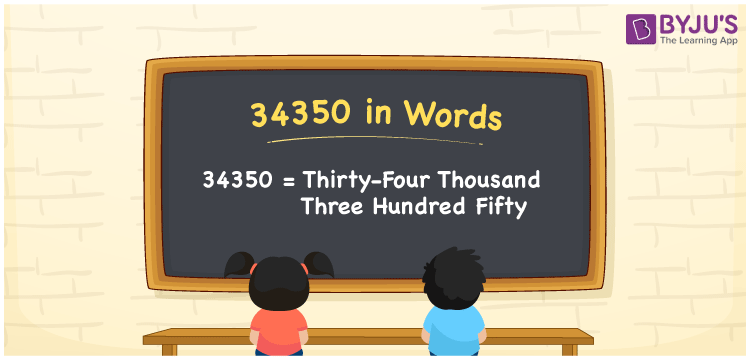# 34350 in Words

34350 in words is written as Thirty-four thousand three hundred fifty. In both the International System of Numerals and the Indian System of Numerals, 34350 is written as Thirty-four thousand three hundred fifty. The number 34350 is a Cardinal Number as it could represent some quantity. For example, “his monthly salary is 34350 rupees”.

 34350 in Words Thirty-four thousand three hundred fifty Thirty-four thousand three hundred fifty in Number 34350

## 34350 in English Words

34350 in English words is read as “Thirty-four thousand three hundred fifty”.## How to Write 34350 in Words?

To write 34350 in words, we shall use the place value chart. In the place value chart, put 3 in the ten thousand, 4 in the thousands, 3 in the hundreds, 5 in the tens and 0 in the ones. Let us make a place value chart to write the number 34350 in words.

 Ten Thousands Thousands Hundreds Tens Ones 3 4 3 5 0

Thus, we can write the expanded form as

3 × Ten Thousand + 4 × Thousand + 3 × Hundred + 5 × Ten + 0 × One

= 3 × 40000 + 4 × 4000 + 3 × 400 + 5 × 40 + 0 × 4

= 30000 + 4000 + 300 +50 + 0

= 34350

= Thirty-four thousand three hundred fifty.

34350 is a natural number, the successor of 34349 and the predecessor of 34351.

34350 in words – Thirty-four thousand three hundred fifty

• Is 34350 an odd number? – No
• Is 34350 an even number? – Yes
• Is 34350 a perfect square number? – No
• Is 34350 a perfect cube number? – No
• Is 34350 a prime number? – No
• Is 34350 a composite number? – Yes

## Frequently Asked Questions on 34350 in Words

Q1

### How to write 34350 in words?

34350 in words is written as Thirty-four thousand three hundred fifty.
Q2

### How to write 34350 in the International and Indian System of Numerals?

In both, the system of numerals, 34350 in words, is written as Thirty-four thousand three hundred fifty.
Q3

### How to write 34350 in a place value chart?

In the place value chart, write 3 in the ten thousand, 4 in the thousands, 3 in the hundreds, 5 in the tens and 0 in the ones, respectively.